# Graphical Solution Of a Linear Equation

## Linear equations in two variable of Class 9

(A) in order to draw the graph of a linear equation in one variable we may follow the following algorithm.

Step I :Obtain the linear equation.

Step II: If the equation is of the form ax = b, a ≠ o, then plot the point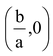and one more point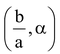when α is any real number. If the equation is of the form ay = b,  a ≠ 0, then plot the point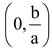and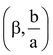where β is any real number.

Step III: Joint the points plotted in step II to obtain the required line.If eq. is in form ax = b then we get a line parallel to Y-axis and if eg. is in form ay = b then we get a line parallel to X-axis.

question . Draw the graph of

(i) 2x + 5 = 0 (ii) 3y - 15 = 0

Solution: (i) Graph of 2x + 5 = 0

On simplifying it we get 2x = –5 ⇒ x = – 5/2

First we plot point A1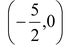& then we plot any other point A2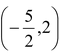on the graph paper, then we join these two points we get required line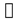as shown in figure below.

(ii) Graph of 3y - 15 = 0

On simplifying it we get 3u = 15 ⇒ y = 15/3  f = 5.

First we plot the point B1 (0, 5) & then we plot any other point B2 (3, 5) on the graph paper, then we join these two points we get required line m as shown in figure.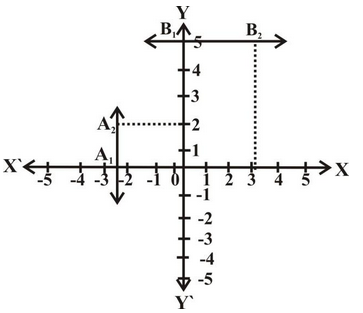NOTE:

(A) A point which lies on the line is a solution of that equation. A point not lying on the line is not a solution of the equation.

(B) In order to draw the graph of a linear equation ax + by + c = 0 may follow the following algorithm.

Step I : Obtain the linear equation ax + by + c = 0.

Step II : Express y in terms of x i.e. y =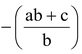or x in terms of y i.e.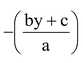.

Step III : Put any two or three values for x or y and calculate the corresponding values of y or x respectively from the expression obtained in Step II. Let we get points as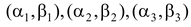.

Step IV : Plot the points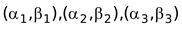on graph paper.

Step V : Joint the pints marked in step IV to obtain. The line obtained is the graph of the equation ax +by + c = 0.

5. Draw the graph of the line x – 2y = 3, from the graph find the coordinate of the point when

(i) x = – 5 (ii) y = 0

Solution: Here given equation is x - 2y = 3.

Solving it for y we get 2y = x - 3 ⇒ y =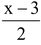Let x = 0, then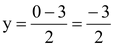x = 3, then y =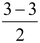= 0,

x = - 2, then y =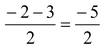Hence we get

 x 0 3 -2 y - 3/2 0 - 5/2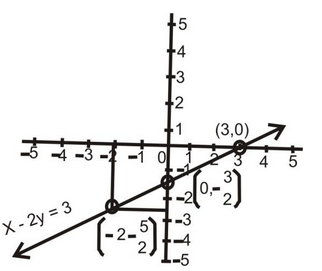Clearly when x = - 5 then y = - 4 and when y = 0 then x = 3.

question . Draw the graphs of the lines represented by the equations x + y = 4 and 2x - y = 2 in the same graph. Also find the coordinate of the point where the two lines intersect.

Solution: Given equations are

x + y = 4 ......(i) & 2x - y = 2 ......(ii)

(i) We have y = 4 - x

 x 0 2 4 y 4 2 0

(ii) We have y = 2x – 2

 x 1 0 3 y 0 –2 4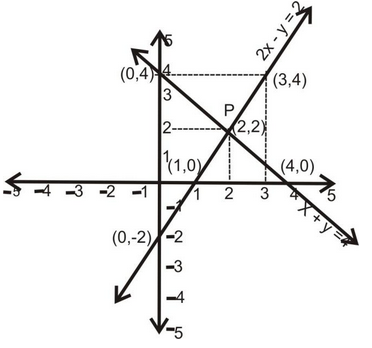By drawing the lines on a graph paper, clearly we can say that P is the point of intersection where coordinates are x = 2, y = 2.